# sfepy.solvers.nls module¶

Nonlinear solvers.

class sfepy.solvers.nls.Newton(conf, **kwargs)[source]

Solves a nonlinear system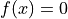using the Newton method.

The solver uses a backtracking line-search on divergence.

Kind: ‘nls.newton’

For common configuration parameters, see Solver.

Specific configuration parameters:

Parameters
i_maxint (default: 1)

The maximum number of iterations.

eps_afloat (default: 1e-10)

The absolute tolerance for the residual, i.e.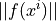.

eps_rfloat (default: 1.0)

The relative tolerance for the residual, i.e.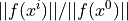.

eps_mode‘and’ or ‘or’ (default: ‘and’)

The logical operator to use for combining the absolute and relative tolerances.

machepsfloat (default: 2.220446049250313e-16)

The float considered to be machine “zero”.

lin_redfloat (default: 1.0)

The linear system solution error should be smaller than (eps_a * lin_red), otherwise a warning is printed.

lin_precisionfloat or None

If not None, the linear system solution tolerances are set in each nonlinear iteration relative to the current residual norm by the lin_precision factor. Ignored for direct linear solvers.

step_red0.0 < float <= 1.0 (default: 1.0)

Step reduction factor. Equivalent to the mixing parameter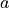: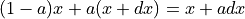ls_onfloat (default: 0.99999)

Start the backtracking line-search by reducing the step, if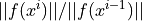is larger than ls_on.

ls_red0.0 < float < 1.0 (default: 0.1)

The step reduction factor in case of correct residual assembling.

ls_red_warp0.0 < float < 1.0 (default: 0.001)

The step reduction factor in case of failed residual assembling (e.g. the “warp violation” error caused by a negative volume element resulting from too large deformations).

ls_min0.0 < float < 1.0 (default: 1e-05)

The minimum step reduction factor.

give_up_warpbool (default: False)

If True, abort on the “warp violation” error.

check0, 1 or 2 (default: 0)

If >= 1, check the tangent matrix using finite differences. If 2, plot the resulting sparsity patterns.

deltafloat (default: 1e-06)

If check >= 1, the finite difference matrix is taken as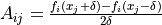.

logdict or None

If not None, log the convergence according to the configuration in the following form: {'text' : 'log.txt', 'plot' : 'log.pdf'}. Each of the dict items can be None.

is_linearbool (default: False)

If True, the problem is considered to be linear.

__annotations__ = {}
__call__(vec_x0, conf=None, fun=None, fun_grad=None, lin_solver=None, iter_hook=None, status=None)[source]

Nonlinear system solver call.

Solves a nonlinear systemusing the Newton method with backtracking line-search, starting with an initial guess.

Parameters
vec_x0array

The initial guess vector.

confStruct instance, optional

The solver configuration parameters,

funfunction, optional

The function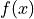whose zero is sought - the residual.

The gradient of- the tangent matrix.

lin_solverLinearSolver instance, optional

The linear solver for each nonlinear iteration.

iter_hookfunction, optional

User-supplied function to call before each iteration.

statusdict-like, optional

The user-supplied object to hold convergence statistics.

Notes

• The optional parameters except iter_hook and status need to be given either here or upon Newton construction.

• Setting conf.is_linear == True means a pre-assembled and possibly pre-solved matrix. This is mostly useful for linear time-dependent problems.

__init__(conf, **kwargs)[source]
__module__ = 'sfepy.solvers.nls'
name = 'nls.newton'
class sfepy.solvers.nls.PETScNonlinearSolver(conf, pmtx=None, prhs=None, comm=None, **kwargs)[source]

Interface to PETSc SNES (Scalable Nonlinear Equations Solvers).

The solver supports parallel use with a given MPI communicator (see comm argument of PETScNonlinearSolver.__init__()). Returns a (global) PETSc solution vector instead of a (local) numpy array, when given a PETSc initial guess vector.

For parallel use, the fun and fun_grad callbacks should be provided by PETScParallelEvaluator.

Kind: ‘nls.petsc’

For common configuration parameters, see Solver.

Specific configuration parameters:

Parameters
methodstr (default: ‘newtonls’)

The SNES type.

i_maxint (default: 10)

The maximum number of iterations.

if_maxint (default: 100)

The maximum number of function evaluations.

eps_afloat (default: 1e-10)

The absolute tolerance for the residual, i.e..

eps_rfloat (default: 1.0)

The relative tolerance for the residual, i.e..

eps_sfloat (default: 0.0)

The convergence tolerance in terms of the norm of the change in the solution between steps, i.e. $||delta x|| < epsilon_s ||x||$

__annotations__ = {}
__call__(vec_x0, conf=None, fun=None, fun_grad=None, lin_solver=None, iter_hook=None, status=None, pmtx=None, prhs=None, comm=None)[source]

Call self as a function.

__init__(conf, pmtx=None, prhs=None, comm=None, **kwargs)[source]
__module__ = 'sfepy.solvers.nls'
name = 'nls.petsc'
class sfepy.solvers.nls.ScipyBroyden(conf, **kwargs)[source]

Interface to Broyden and Anderson solvers from scipy.optimize.

Kind: ‘nls.scipy_broyden_like’

For common configuration parameters, see Solver.

Specific configuration parameters:

Parameters
methodstr (default: ‘anderson’)

The name of the solver in scipy.optimize.

i_maxint (default: 10)

The maximum number of iterations.

alphafloat (default: 0.9)

See scipy.optimize.

Mfloat (default: 5)

See scipy.optimize.

f_tolfloat (default: 1e-06)

See scipy.optimize.

w0float (default: 0.1)

See scipy.optimize.

__annotations__ = {}
__call__(vec_x0, conf=None, fun=None, fun_grad=None, lin_solver=None, iter_hook=None, status=None)[source]

Call self as a function.

__init__(conf, **kwargs)[source]
__module__ = 'sfepy.solvers.nls'
name = 'nls.scipy_broyden_like'
set_method(conf)[source]

Verify the correctness of the tangent matrix as computed by fun_grad() by comparing it with its finite difference approximation evaluated by repeatedly calling fun() with vec_x0 items perturbed by a small delta.

sfepy.solvers.nls.conv_test(conf, it, err, err0)[source]

Nonlinear solver convergence test.

Parameters
confStruct instance

The nonlinear solver configuration.

itint

The current iteration.

errfloat

The current iteration error.

err0float

The initial error.

Returns
statusint

The convergence status: -1 = no convergence (yet), 0 = solver converged - tolerances were met, 1 = max. number of iterations reached.